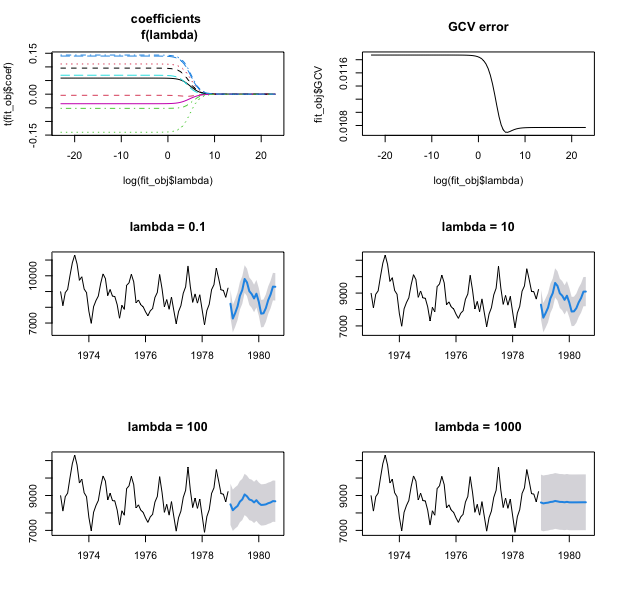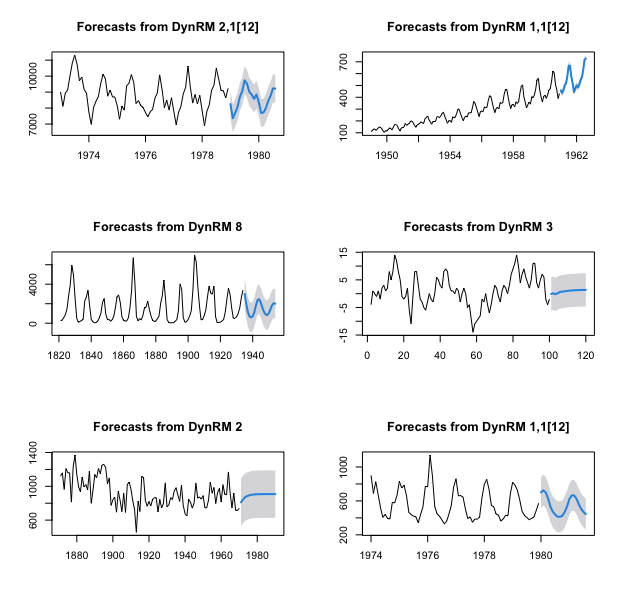Last week I presented ahead, an R package for univariate and multivariate time series forecasting. In particular, the function dynrmf was introduced for univariate time series, with examples of Random Forest and Support Vector Machines fitting functions (fitting and predicting through fit_func and predict_func arguments of dynrmf). First things first, here’s how to install R package ahead:

• 1st method: from R-universe

In R console:

  options(repos = c(
techtonique = 'https://techtonique.r-universe.dev',
CRAN = 'https://cloud.r-project.org'))


• 2nd method: from Github

In R console:

  devtools::install_github("Techtonique/ahead")


Or

  remotes::install_github("Techtonique/ahead")


In version 0.2.0 of ahead, Ridge regression is the default fitting function for dynrmf. Let’s see how it works:

library(datasets)

# We start by a demo of ahead's Ridge regression implementation on random tabular data
set.seed(123)
n <- 100 ; p <- 10
X <- matrix(rnorm(n * p), n, p)
y <- rnorm(n)

# default behavior for ahead::ridge: a sequence of 100 regularization parameters lambdas is provided

# plot
par(mfrow=c(3, 2))
# regression coefficients (10) as a function of log(lambda)
matplot(log(fit_obj$lambda), t(fit_obj$coef), type = 'l',  main="coefficients \n f(lambda)")
# Generalized Cross Validation (GCV) error as a function of log(lambda)
plot(log(fit_obj$lambda), fit_obj$GCV, type='l', main="GCV error")
# dynrmf with different values of the regularization parameter lambda
# ahead::ridge is provided as default fit_func, you can print(head(ahead::dynrmf))
plot(ahead::dynrmf(USAccDeaths, h=20, level=95, fit_params=list(lambda = 0.1)), main="lambda = 0.1")
plot(ahead::dynrmf(USAccDeaths, h=20, level=95, fit_params=list(lambda = 10)), main="lambda = 10")
plot(ahead::dynrmf(USAccDeaths, h=20, level=95, fit_params=list(lambda = 100)), main="lambda = 100")
plot(ahead::dynrmf(USAccDeaths, h=20, level=95, fit_params=list(lambda = 1000)), main="lambda = 1000")As demonstrated in the previous code snippet, you can try different values of the regularization parameter lambda, and see how ahead’s performance is influenced by each one of your choices. However, if you do not choose a regularization parameter $$\lambda$$, the one that minimizes Generalized Cross Validation (GCV) error is automatically (automatically, yes, but not pretending that this will always guarantee the best out-of-sample accuracy) picked internally, on a grid of 100 values. In the examples below of dynrmf, the $$\lambda$$ that minimizes Generalized Cross Validation (GCV) error is picked internally :

par(mfrow=c(3, 2))
# nothing else required, default is Ridge regression with minimal GCV lambda